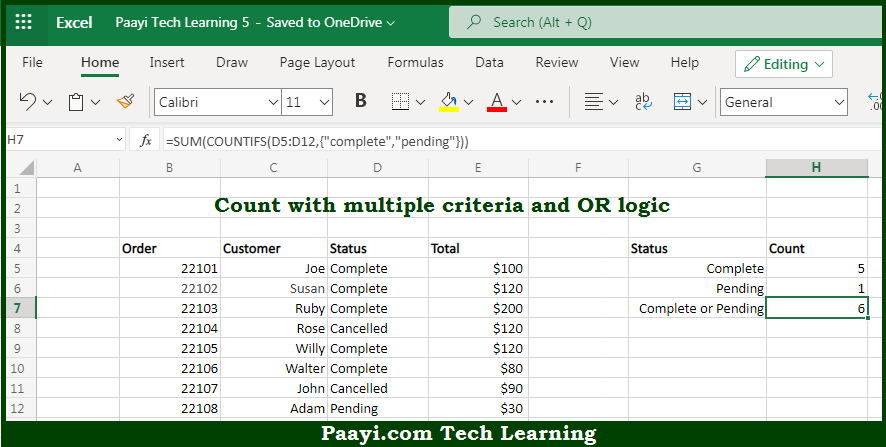# Learn How to COUNTIFS With Multiple Criteria and OR Logic in Microsoft Excel

Written by | 0 Comments | 404 Views

In this article, you will learn how to COUNT various things in Microsoft Excel using a single or combination of functions and its purpose. You will also get to know how to COUNTIFS With Multiple Criteria and OR Logic and see the generic formula.

COUNTIFS With Multiple Criteria and OR Logic in Microsoft Excel

The main purpose of this formula is to count with multiple criteria and OR logic. Here we will learn how to count with multiple criteria using OR logic with given criteria in Microsoft Excel. That implies, with the help of a formula based on the COUNTIFS function you can able to count with multiple criteria using OR logic. So, with the help of this formula, you can able to count with multiple criteria and OR logic.

General Formula to COUNTIFS With Multiple Criteria and OR Logic

=SUM(COUNTIFS(range,{"red","blue","green"}))

The Explanation for the COUNTIFS With Multiple Criteria and OR LogicSo we know that with the help of the given formula above you can able to count with multiple criteria and OR logic. Here we will learn how to count with multiple criteria using OR logic with given criteria in Microsoft Excel. As we know that the By default, the COUNTIFS function applies AND logic. When you supply multiple conditions, all conditions must match in order to generate a count. One solution is to supply multiple criteria in an array constant as shown in the example above. So, with the help of this formula, you can able to count with multiple criteria using OR logic.